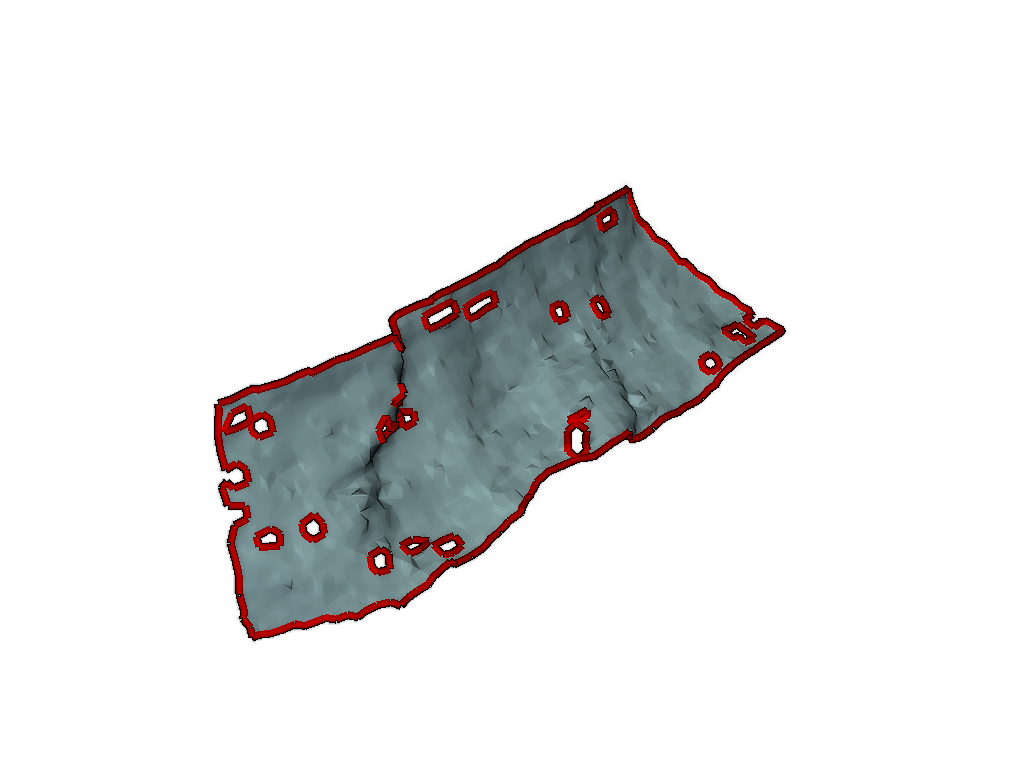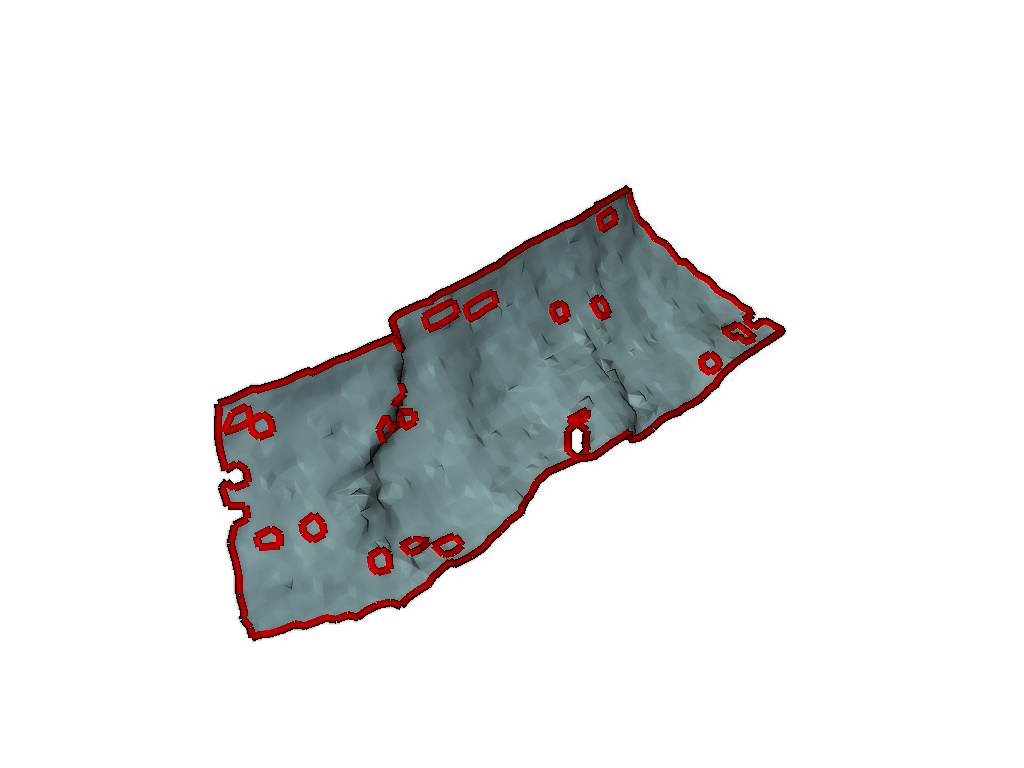# Partial Fill Holes#

This example fills all but the largest holes in a planar mesh.

```# sphinx_gallery_thumbnail_number = 1
import numpy as np
from pymeshfix import MeshFix
from pymeshfix._meshfix import PyTMesh
from pymeshfix.examples import planar_mesh
import pyvista as pv
```

plot the holes on the original mesh

```orig_mesh = pv.read(planar_mesh)
# orig_mesh.plot_boundaries()

meshfix = MeshFix(orig_mesh)
holes = meshfix.extract_holes()

# Render the mesh and outline the holes
plotter = pv.Plotter()
plotter.enable_eye_dome_lighting()  # helps depth perception
_ = plotter.show()
```This example uses the lower level C interface to the TMesh object.

```mfix = PyTMesh(False)  # False removes extra verbose output

# Fills all the holes having at at most 'nbe' boundary edges. If
# 'refine' is true, adds inner vertices to reproduce the sampling
# density of the surroundings. Returns number of holes patched.  If
# 'nbe' is 0 (default), all the holes are patched.
mfix.fill_small_boundaries(nbe=100, refine=True)
```
```16
```

Convert back to a pyvista mesh

```vert, faces = mfix.return_arrays()
triangles = np.empty((faces.shape, 4), dtype=faces.dtype)
triangles[:, -3:] = faces
triangles[:, 0] = 3

mesh = pv.PolyData(vert, triangles)
```

Plot the repaired mesh along with the original holes Note: It seems there is a limit to the repair algorithm whereby some of the holes that include only a single point are not filled. These boundary holes are not detected by VTK’s hole repair algorithm either.

```plotter = pv.Plotter()